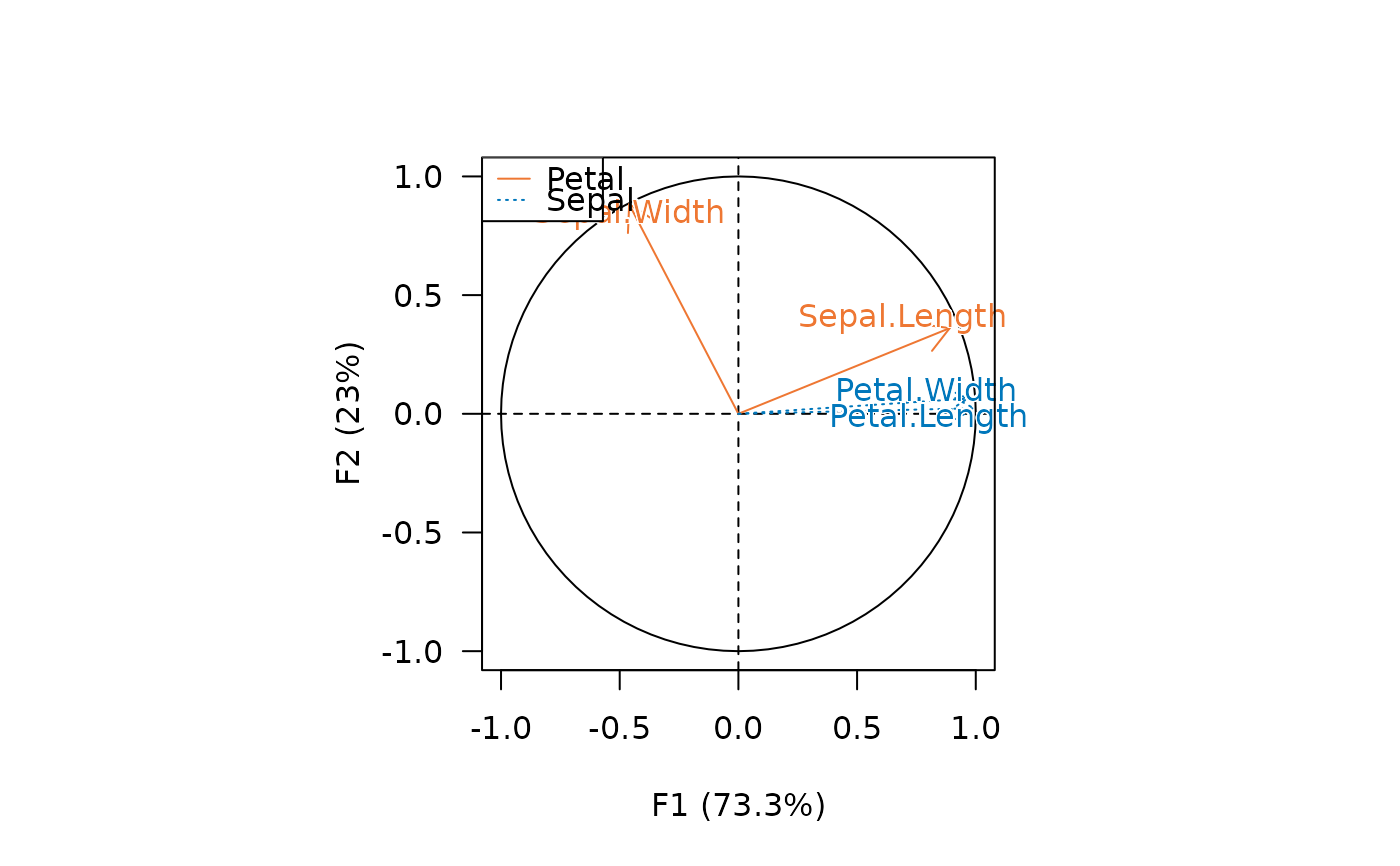Plots row/individual principal coordinates.

## Usage

viz_individuals(x, ...)

viz_rows(x, ...)

# S4 method for MultivariateAnalysis
viz_rows(
x,
axes = c(1, 2),
active = TRUE,
sup = TRUE,
labels = FALSE,
highlight = NULL,
main = NULL,
sub = NULL,
panel.first = NULL,
panel.last = NULL,
...
)

# S4 method for BootstrapCA
viz_rows(x, axes = c(1, 2), ...)

# S4 method for PCA
viz_individuals(
x,
axes = c(1, 2),
active = TRUE,
sup = TRUE,
labels = FALSE,
highlight = NULL,
main = NULL,
sub = NULL,
panel.first = NULL,
panel.last = NULL,
...
)

## Arguments

x

A CA or PCA object.

...

Further graphical parameters (see details).

axes

A length-two numeric vector giving the dimensions to be plotted.

active

A logical scalar: should the active observations be plotted?

sup

A logical scalar: should the supplementary observations be plotted?

labels

A logical scalar: should labels be drawn?

highlight

A vector specifying the information to be highlighted. If NULL (the default), no highlighting is applied. It will only be mapped if at least one graphical parameters is explicitly specified (see examples). If a single character string is passed, it must be one of "observation", "mass", "sum", "contribution" or "cos2" (see augment()). Any unambiguous substring can be given.

main

A character string giving a main title for the plot.

sub

A character string giving a subtitle for the plot.

panel.first

An an expression to be evaluated after the plot axes are set up but before any plotting takes place. This can be useful for drawing background grids.

panel.last

An expression to be evaluated after plotting has taken place but before the axes, title and box are added.

## Value

viz_*() is called for its side-effects: it results in a graphic being displayed. Invisibly returns x.

## Details

Commonly used graphical parameters are:

pch

A vector of plotting characters or symbols. This can either be a single character or an integer code for one of a set of graphics symbols.

cex

A numerical vector giving the amount by which plotting characters and symbols should be scaled relative to the default.

col

The colors for lines and points. Multiple colors can be specified so that each point can be given its own color.

bg

The background color for the open plot symbols given by pch = 21:25.

## Note

Be careful: graphical parameters are silently recycled.

Other plot methods: biplot(), screeplot(), viz_contributions(), viz_variables(), viz_wrap, wrap

N. Frerebeau

## Examples

## Load data
data("iris")

## Compute principal components analysis
X <- pca(iris, scale = TRUE)
#> 1 qualitative variable was removed: Species.

## Plot individuals
viz_individuals(X, panel.last = graphics::grid())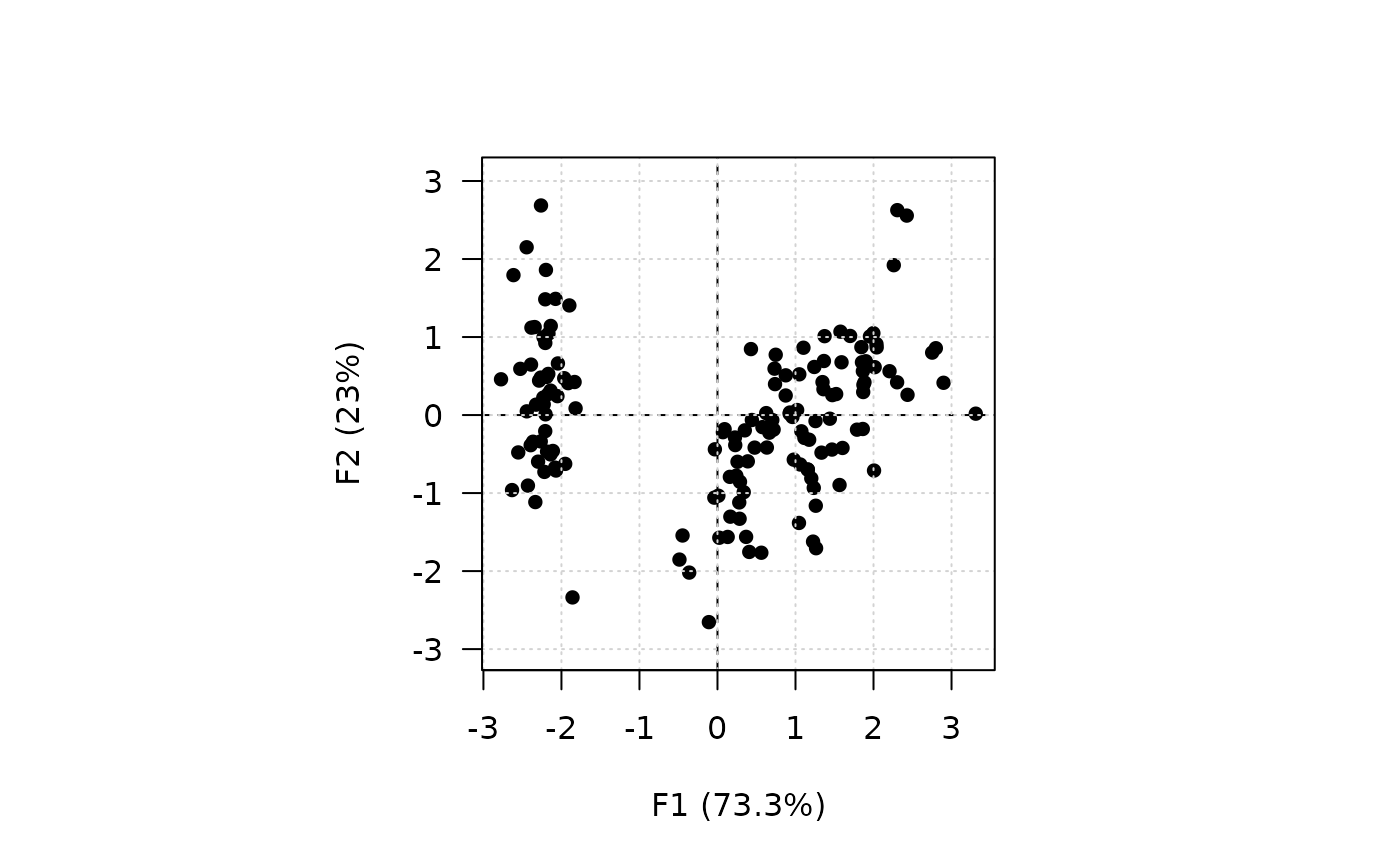## Plot variables
viz_variables(X, panel.last = graphics::grid())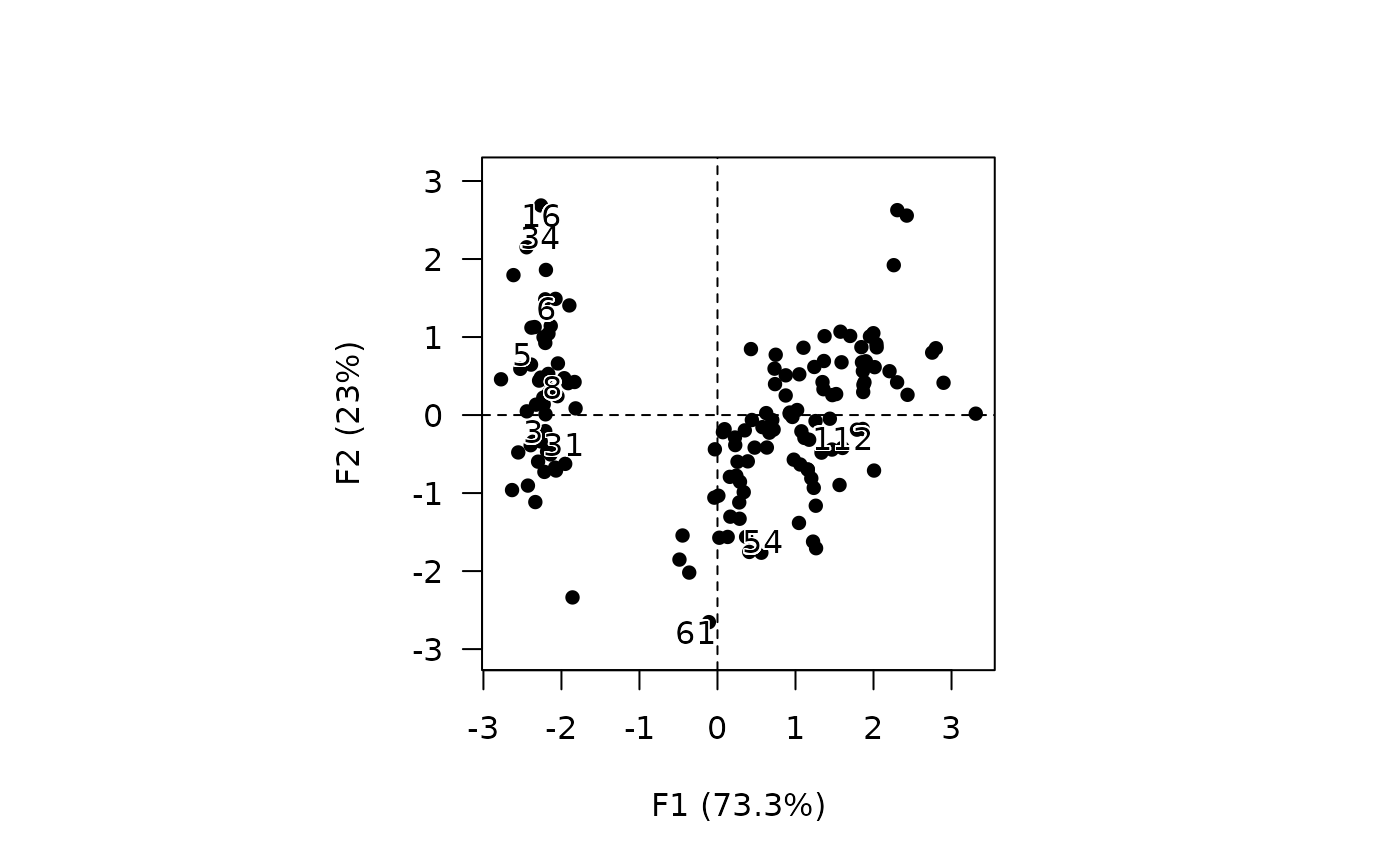## Graphical parameters
## Continuous values
viz_individuals(X, highlight = iris$Petal.Length, pch = 16)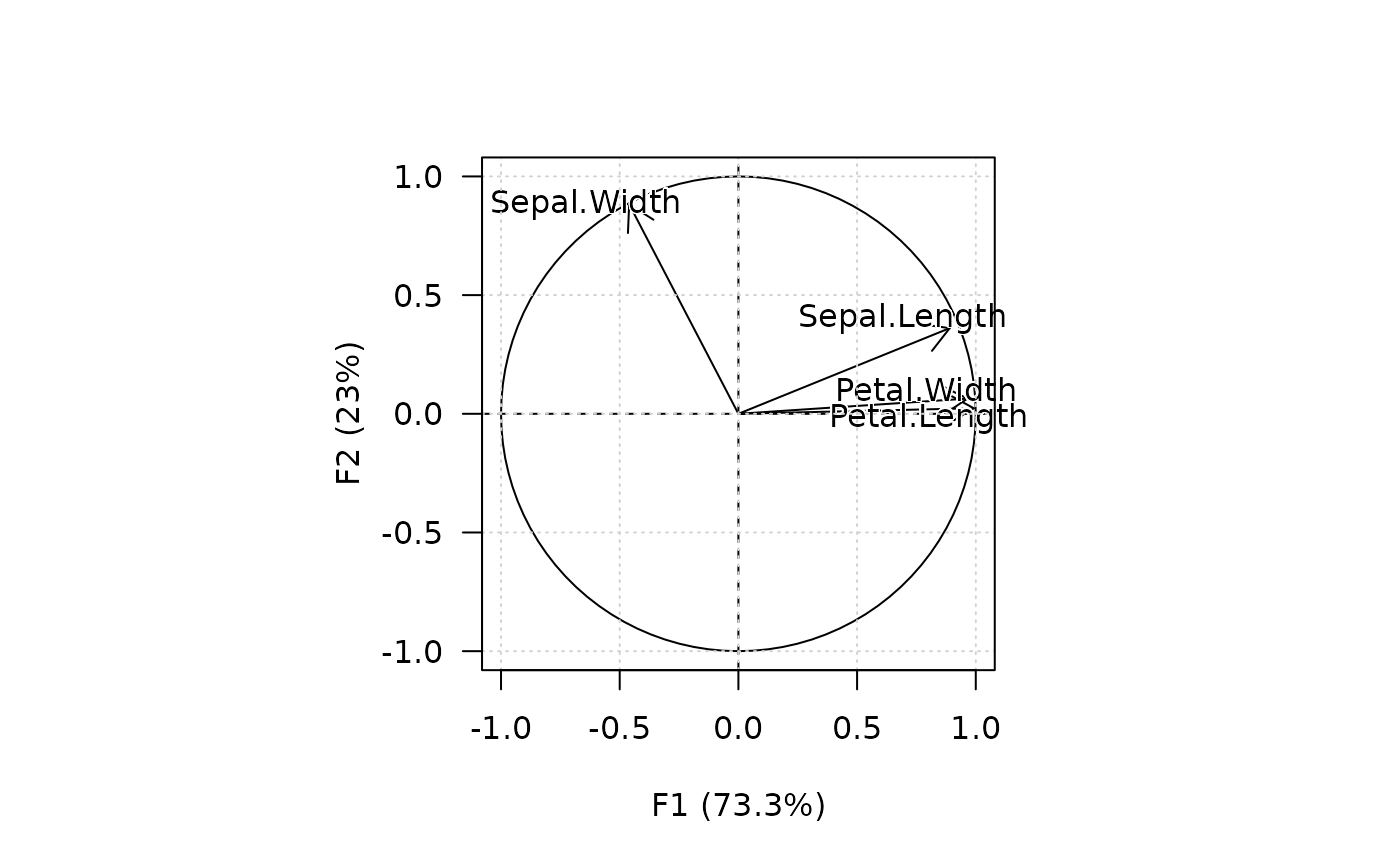viz_individuals(X, highlight = iris$Petal.Length, pch = 16,
col = grDevices::hcl.colors(12, "RdPu"))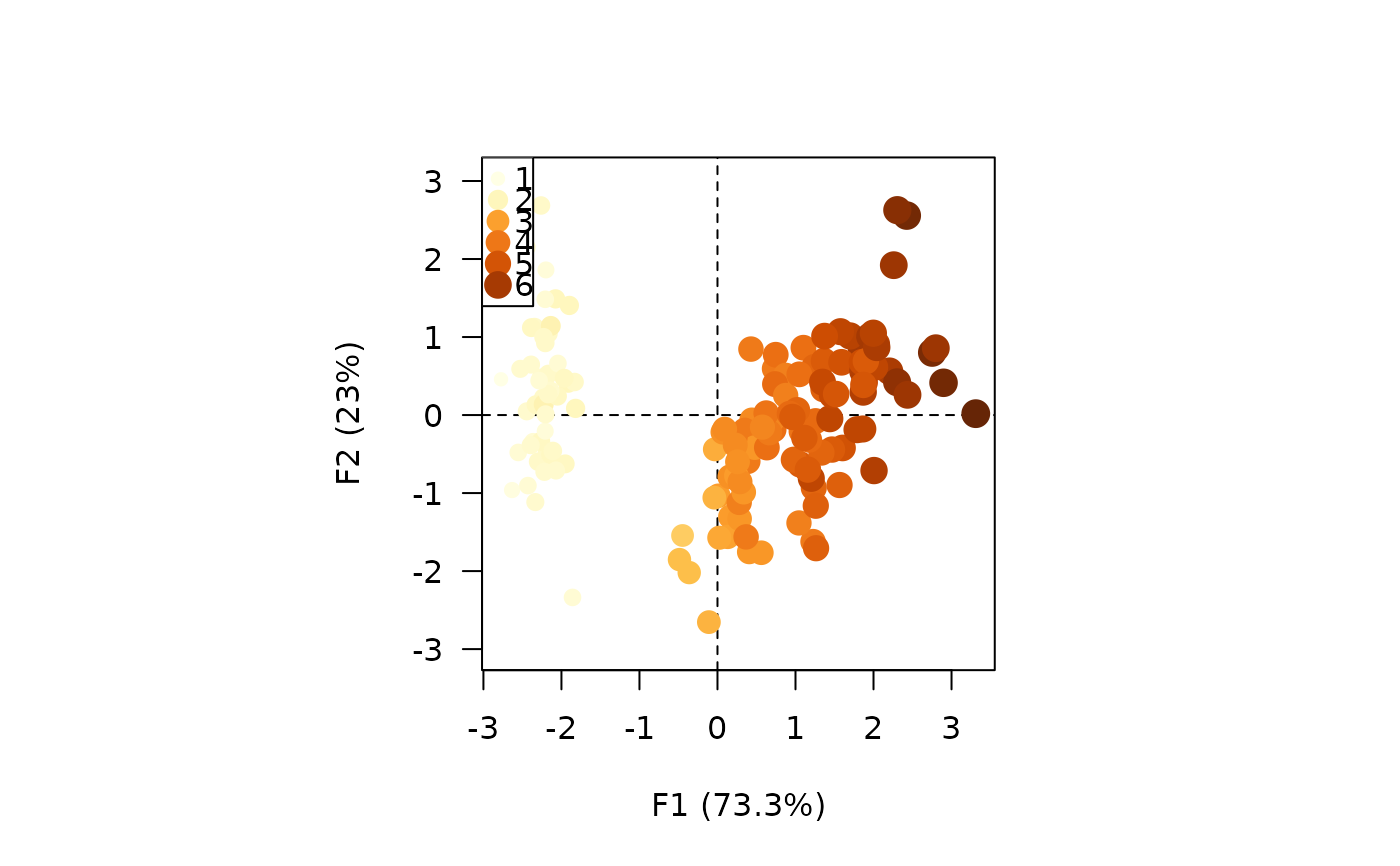viz_individuals(X, highlight = iris$Petal.Length, pch = 16, col = grDevices::hcl.colors(12, "RdPu"), cex = c(1, 2))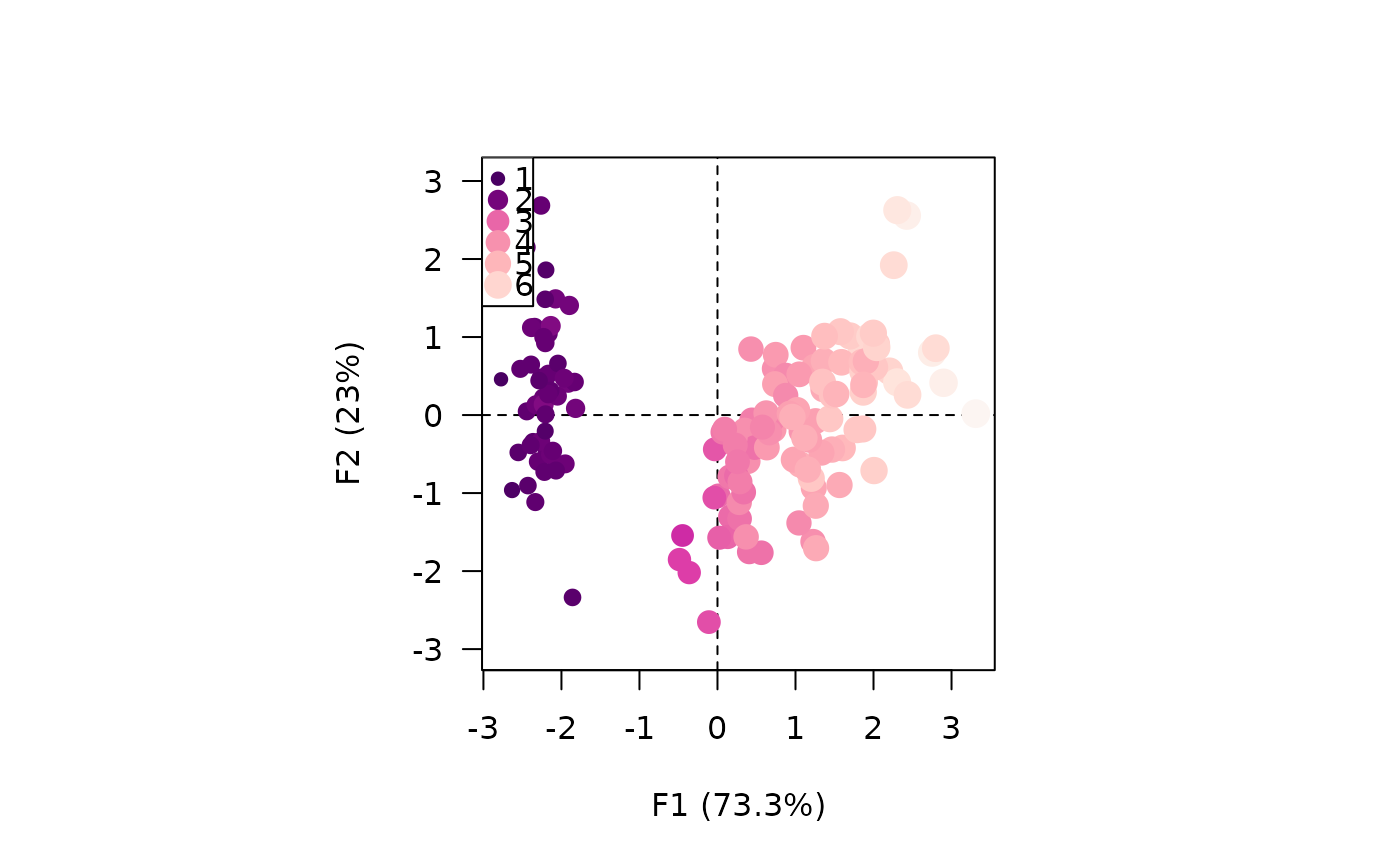viz_variables(X, highlight = "contribution", col = grDevices::hcl.colors(12, "BluGrn", rev = TRUE), lwd = c(1, 5))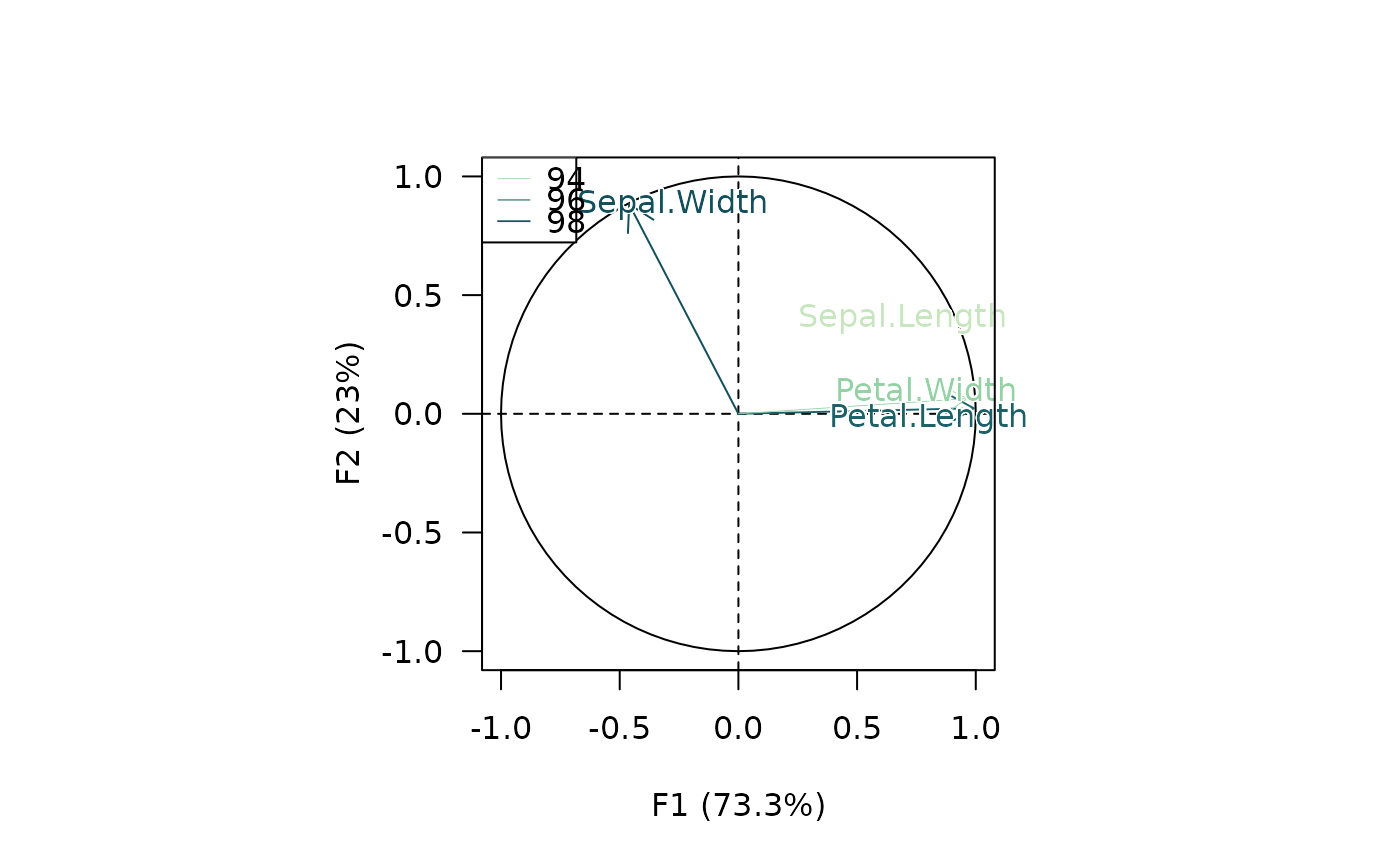## Discrete values viz_individuals(X, highlight = iris$Species, pch = 21:23)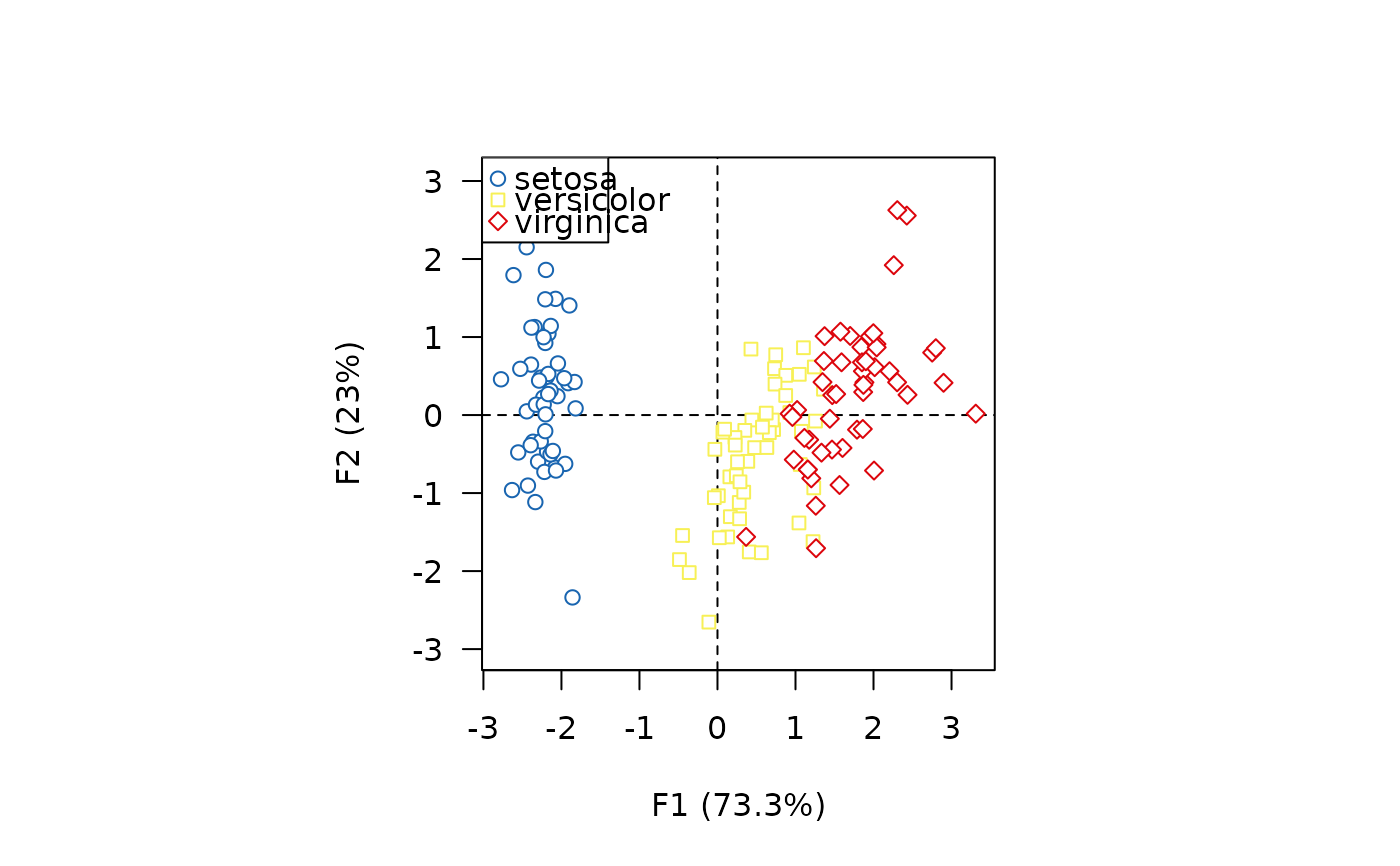viz_individuals(X, highlight = iris\$Species, pch = 21:23,
bg = c("#004488", "#DDAA33", "#BB5566"),
col = "black")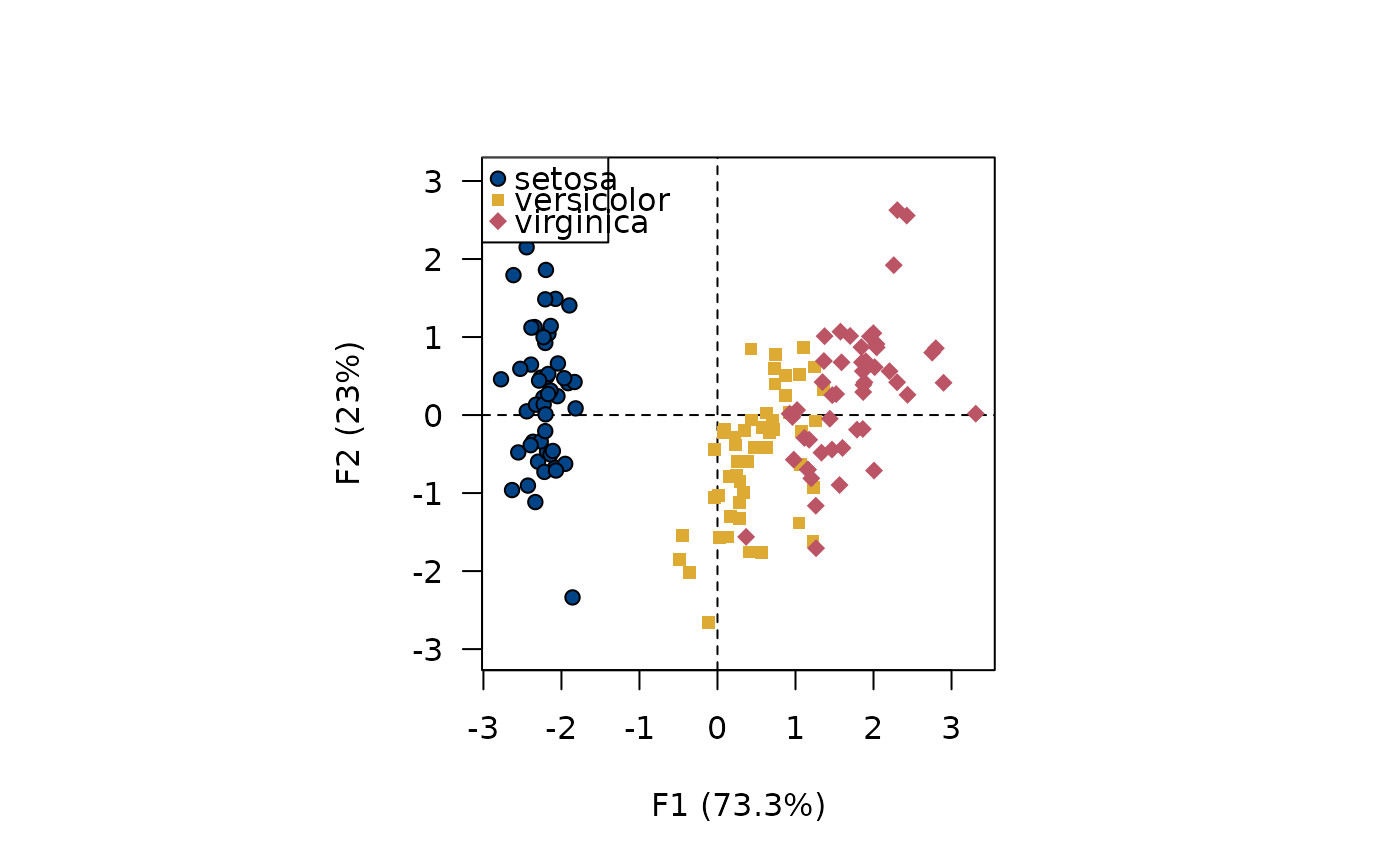viz_variables(X, highlight = c("Petal", "Petal", "Sepal", "Sepal"),
col = c("#EE7733", "#0077BB"),
lty = c(1, 3))